Impact Factor 3.648 | CiteScore 3.99
More on impact ›

# Frontiers in Neuroscience

## Original Research ARTICLE

Front. Neurosci., 30 September 2010 | https://doi.org/10.3389/fnins.2010.00172

# Effect of sampling frequency on the measurement of phase-locked action potentials

• Department of Biology, University of Maryland, College Park, MD, USA

Phase-locked spikes in various types of neurons encode temporal information. To quantify the degree of phase-locking, the metric called vector strength (VS) has been most widely used. Since VS is derived from spike timing information, error in measurement of spike occurrence should result in errors in VS calculation. In electrophysiological experiments, the timing of an action potential is detected with finite temporal precision, which is determined by the sampling frequency. In order to evaluate the effects of the sampling frequency on the measurement of VS, we derive theoretical upper and lower bounds of VS from spikes collected with finite sampling rates. We next estimate errors in VS assuming random sampling effects, and show that our theoretical calculation agrees with data from electrophysiological recordings in vivo. Our results provide a practical guide for choosing the appropriate sampling frequency in measuring VS.

## Introduction

Information coding via synchronized neural activity is a common feature in the nervous system. Various types of neurons encode temporal information by phase-locked spiking activities (Carr and Friedman, 1999). Phase-locking is most widely seen in the auditory system, including auditory nerves or auditory brainstem neurons in dogs (Goldberg and Brown, 1969), redwing blackbirds (Sachs and Sinnott, 1978), cats (Johnson, 1980; Joris et al., 1994), guinea-pigs (Palmer and Russell, 1986), songbirds (Gleich and Narins, 1988), pigeons (Hill et al., 1989), chicks (Salvi et al. 1992), owls (Köppl, 1997), emus (Manley et al., 1997), geckos (Sams-Dodd and Capranica, 1994), caimans and alligators (Smolders and Klinke, 1986; Carr et al., 2009), and auditory cortex neurons in cats (Eggermont and Smith, 1995). Apart from the auditory system, phase-locking has also been found in electrosensory lateral line lobe neurons in weakly electric fish (Kawasaki and Guo, 1996), Mauthner cells in teleosts (Weiss et al., 2009), frog mechanoreceptor afferents (Ogawa et al., 1981), locust olfactory system (Stopfer et al., 2003), rat barrel cortex (Ewert et al., 2008), cat visual cortex (Gray and Singer, 1989), and rat hippocampal place cells (Harris et al., 2002; Diba and Buzsáki, 2008; Mizuseki et al., 2009).

In electrophysiological experiments, action potentials are detected from intra- or extracellular potentials and a sequence of spikes (“spike train”) is obtained. In most cases, the internal clock of the recording system determines the temporal resolution of data acquisition and therefore spike timing data can be obtained only with finite temporal accuracy (Figure 1). Collected spike timing could be shifted as much as the length of the clock cycle or the “sampling window.” Any quantity or metric derived from spike timing information is more or less subject to this temporal uncertainty. In this paper, we refer to the error emerged from finite temporal sampling resolution as “temporal sampling error.” Theoretically (and intuitively), sampling rate, which is the reciprocal of the length of the sampling window, should be as high as possible to obtain precise spike timing data. However, in practice, sampling rate cannot be set arbitrarily high because of costs and technical limitations. Thus any spike timing calculation is subject to errors associated with sampling.

FIGURE 1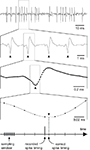Figure 1. Recorded spike waveforms and the effect of sampling window. Since sampling windows have finite lengths, recorded spike timing can be shifted within the length of each sampling window. Filled circles in the third and fourth panels indicate sample points. Filled triangles indicate spike occurrence. In this figure, a peak detector is used to discriminate spikes. Note that other spike detection algorithms, such as threshold crossing detection, are also subject to temporal sampling errors.

Phase-locking, or periodic increase in spike discharge rate at a certain phase of the reference stimulus, is often quantified by the metric called vector strength (VS) (Goldberg and Brown, 1969). The mean vector (X,Y) of a spike train is calculated as: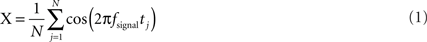and,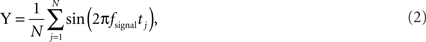where fsignal is the reference signal frequency, tj is the timing of the j-th spike, N is the total number of spikes. VS, or the length of the mean vector, is calculated as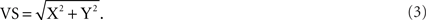By definition, VS takes values between 0 and 1 (Fisher, 1993). A VS of 1 means that all the spikes occurred in a certain phase of the signal (i.e., perfect phase-locking) and a VS of 0 implies that the spike train has no phase preference for the reference signal. Since VS is a quantity derived from spike timing information, it can be substantially affected by the temporal sampling error. How high a sampling rate is high enough to obtain an accurate measure of VS? How robust a measure is VS when sampling rate is not ideally high? In this technical note, we derive theoretical upper and lower bounds for errors in VS calculated from spikes collected with finite sampling rates. We also calculate errors in VS using an assumption of random sampling effects, and compare our theoretical estimation with data from in vivo recordings. Our results provide a practical guideline for determining the appropriate size of the sampling window in measuring VS.

## Materials and Methods

### In vivo Recordings of Auditory Brainstem Neurons

Data from auditory brainstem neurons in barn owls, chicks and American alligators were used to assess the effect of sampling on the calculation of VS. Animal husbandry and experimental protocols were approved by the Animal Care and Use Committee of the University of Maryland, the Regierung von Oberbayern (Germany), the University of Sydney Animal Ethics Committee, and/or the Marine Biological Laboratory (Woods Hole, MA, USA). Detailed procedures for surgery, stereotaxis, acoustic stimulus generation, and data collection have been provided by Carr and Köppl (2004) for owls, Köppl and Carr (2008) for chicks, and Carr et al. (2009) for alligators. In brief, animals were anesthetized and placed in a sound-attenuating chamber. Body temperature was maintained by a feedback-controlled heating blanket. An electrocardiogram was recorded via needle electrodes placed in the muscles of legs and/or wings to monitor muscle potentials and the heart beat. The head was held in a constant position by gluing a stainless steel head post and the skull was opened to expose the cerebellum. If necessary, a portion of the cerebellum was aspirated to expose the dorsal surface of the brainstem. Recordings were made with tungsten (2–20 MΩ) or glass electrodes (5–100 MΩ).

Custom-written software (xdphys, Caltech, CA, USA) was used for controlling acoustic stimuli and collecting data together with the TDT2 signal-processing system (Tucker Davis Technology, TDT, Gainesville, FL, USA). Acoustic stimuli were passed through a D/A converter (TDT DD1), filtered (TDT FT6-2), attenuated (TDT PA4), impedance-matched (TDT HB4) and delivered to the animal by earphones placed into the ear canals. Sound pressure levels were calibrated before recordings using built-in miniature microphones (Knowles EM3068, Itasca, IL, USA). Responses to acoustic stimuli were continuously monitored until the electrode reached the cochlear nuclei in the auditory brainstem (nucleus magnocellularis, NM; or nucleus laminaris, NL). After isolating a single unit, characteristic frequency (CF) and response threshold at CF were determined (Köppl and Carr, 2003). To measure the degree of phase-locking, continuous tones at or near the CF were presented with an intensity of 20 dB above the threshold. Signals from the electrode were amplified and filtered by a custom-built headstage and amplifier and passed through an A/D converter (TDT DD1), a threshold discriminator (TDT SD1) with an event timer (TDT ET1) and fed to the computer. In about half of the recordings, extracellular potential waveforms were stored to the computer and later analyzed. In other cases, only spike timing data generated by the level detector (TDT SD1) were stored. Both the potential waveforms and the spike timing data were digitized and stored at a sampling rate of 48077 Hz.

### Data Analysis, Down-Sampling, and Calculation of Vector Strengths

Custom-written Matlab (MathWorks, Natick, MA, USA) scripts were used for data analysis. For units with potential waveform data, spike timings tj were calculated by peak detection (Figure 1) and VS was calculated according to Eqs. 1–3. For units without potential waveforms, stored spike timing data (which was generated by the threshold discriminator) was used to calculate VS. Note that no significant difference between data with and without potential waveforms was found in the results shown in Section “Examples From In Vivo Recording.” For each single unit, timing data from 400 to 10000 action potentials were stored. For Figure 6, we used timing data of 400 spikes from each unit recording to calculate VS.

To quantify the effect of sampling rate on VS calculation, potential waveforms or spike timing data were down-sampled with various sampling frequencies fsample. Peaks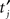of each downsampled waveform were detected and VS of the spike train was computed. For a unit without a stored waveform, downsampled spike timingwas assigned by shifting each spike time tj to the nearest sampling point after tj and VS was calculated. In order to test significance of the phase preference, we calculated the significance probability for VS of each spike train by P = exp(−N(VS)2) with N being the number of spikes (Fisher, 1993). All the single unit data used in our analysis satisfy VS > 0.2 and N > 400, yielding P < 1.1 × 10−7.

## Results

In this section, we evaluate the effect of temporal sampling error on VS calculation by deriving the lower and upper bounds for VS, examining expected error in VS, and comparing our theoretical calculation with physiologically recorded data in vivo.

### Upper and Lower Bounds of Vector Strength

In this subsection, we derive the theoretical upper and lower bounds of VS values with temporal sampling errors. We assume, for theoretical simplicity, that a sufficiently large number of spikes are collected and that the von Mises distribution (Fisher, 1993) can properly approximate the phase histogram of the spike trains.

Let g(x) be a periodic function with a period of 2π and be normalized as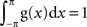. The mean vector (X,Y) of the function g(x) is defined as: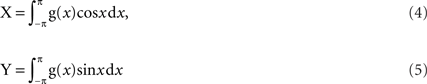and the VS is: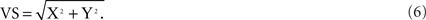The von Mises distribution is defined as: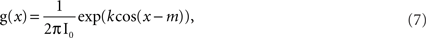where k and m are the parameters determining the concentration and the mean phase, respectively. I0 is the modified Bessel function of order zero satisfying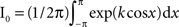and thus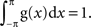By assuming m = 0 without any loss of generality, VS with the von Mises distribution can simply be calculated as: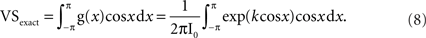The subscript “exact” means that no temporal sampling error is incorporated in this calculation. An example is given in Figure 2A.

FIGURE 2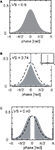Figure 2. Theoretical upper and lower bounds of VS. (A) Example of the von Mises distribution with a concentration parameter k = 1.5157, mean direction m = 0 (rad) and vector strength VS = 0.6. (B) Increase in estimated VS due to sampling biased toward the mean direction. The sharp peak at 0 (rad) indicates a delta function, or dense concentration of the unevenly sampled distribution. Width of sampling window W = 0.2π (sampling ratio R = 0.1). (C) Decrease in estimated VS due to biased sampling opposite to the mean direction. The peaks at ±π (rad) indicate delta functions, or dense concentration of the unevenly sampled distribution. Width of sampling window = 0.2π (sampling ratio R = 0.1). Since delta functions cannot be drawn exactly, bars with a bin width of π/50 (rad) were drawn instead (B,C). Inset in (B) shows the peak of the binned delta function.

As discussed in the previous subsection, collected spike timing can be shifted within the length of the sampling window T = 1/fsample. This temporal sampling error corresponds to a maximum phase error of ±πR. In the following text, R = fsignal/fsample is referred to as the “sampling ratio.” The theoretical upper bound of the VS is obtained by assuming that all the spike timings are shifted in a biased fashion toward the direction of the mean phase of the original distribution to increase the value of VS (Figure 2B). In this case, the length of the mean vector of the shifted spike train is calculated as: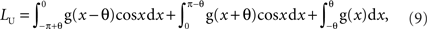where θ = πR = πfsignal/fsample. The first, second, and third terms denote the contribution of the probability distributions on (−π,0), the distribution on (0,π) and the distribution concentrated at phase 0, respectively. The upper bound of VS is: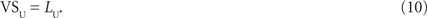The lower bound of VS can be obtained similarly but assumes that all the spike timings are shifted toward the opposite direction of the mean phase of the original distribution to decrease the value of VS (Figure 2C). In this case, the length of the mean vector of the shifted spike train is calculated as: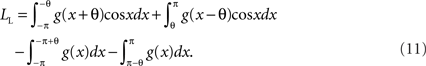The first, second, third, and fourth terms denote the contribution of the probability distributions on (−π,0), the distribution on (0,π), the distribution concentrated at phase −π, and the distribution concentrated at phase π, respectively. In contrast to the upper bound LU, the value of LL can be less than 0, since the “length” here is calculated with respect to the direction of the mean phase of the original distribution. A negative value of LL means that the mean vector of the shifted spike train lies in the opposite direction of the original direction and in such a case VS can take an arbitrary value between 0 and VSexact. Therefore we obtain the lower bound of VS as: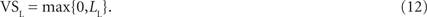The upper and lower bounds for five VS values ranging from 0.1 to 0.9 are shown in Figure 3 (dashed lines). The horizontal axis is the sampling ratio R = fsignal/fsample. When the sampling ratio increases to 1, the upper bound of VS approaches to 1 and the lower bound to 0. This means that we cannot obtain a good estimate of VS if the sampling rate is as low as the reference stimulus frequency. Since the upper and lower bounds depend on VSexact, we calculated the theoretical “maximum error” as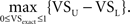Maximum VS errors calculated for several sampling rates are shown in Table 1. For R < 0.1, the maximum error is almost linear with R.

FIGURE 3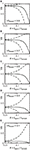Figure 3. (A–E) Estimated VS plotted against sampling ratio R = fsignal/fsample. Dashed lines indicate the theoretical upper and lower bounds of VS calculated from the von Mises distribution. Solid lines show vector strength calculated with an assumption of random sampling errors. Exact vector strengths VSexact of 0.9 (A), 0.7 (B), 0.5 (C), 0.3 (D), 0.1 (E) were used.

TABLE 1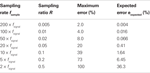Table 1. Errors in VS calculation. Maximum errors are obtained from the theoretical upper and lower bounds for VS. Expected errors are calculated as 1 − sinπRR with an assumption of random sampling errors (see text).

### Expected Error of Vector Strength

In the previous section, we obtained the upper and lower bounds of VS, assuming the von Mises distribution. Although these upper and lower bounds are of theoretical importance, it is practically unlikely that sampling is totally biased toward the direction where these limits are attained. In this section, we derive another estimate for error in VS by adopting the more natural assumption that collected spike timing is jittered randomly within the sampling window. Generally, this random sampling jitter flattens the spike distribution. Figure 4 shows examples of narrow (A), wide (B) and extremely wide sampling windows (C). Note that the length of sampling window (=1/fsample) is converted to the length of the window function (=2πfsignal/fsample, see next paragraph for detail). If the sampling window is small (or equivalently, if the sampling rate is high) compared to the reference signal, the effect of temporal sampling error is limited (Figure 4A). If the sampling rate is equal to the signal frequency, the temporal sampling error totally hides the temporal structure of the spike trains (Figure 4C).

FIGURE 4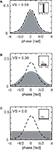Figure 4. Change in the shape of distribution and decrease in estimated VS due to random sampling error. (A) Sampling window width W = 0.2π, sampling ratio R = 0.1 (i.e., fsample = 10 × fsignal). (B) W = 1.0π, R = 0.5 (i.e., fsample = 2 × fsignal). (C) W = 2.0π, R = 1.0 (i.e., fsample = fsignal). Dashed lines indicate the original von Mises distribution with a VS of 0.6 (as shown in Figure 2A), while gray areas show windowed distributions. Inset figures show window functions w(x).

Let g(x) be a periodic function with a period of 2π and be normalized asIn the following derivation, we do not need to assume any particular shape for g(x). Only a sufficiently large number of spikes are assumed to be collected to form the distribution function g(x). Since a spike occurred at phase x is assumed to be randomly shifted within the range of ±θ (θ= πR = πfsignal/fsample), the distribution function h(x) of sampled spikes (Figure 4, gray areas) can be obtained as a convolution of the original distribution function g(x) (Figure 4, dashed lines) and a window function w(x) (Figure 4, insets). Precisely,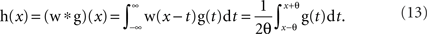The window function w(x) = 1/2θ (−θ < x < θ) and = 0 (otherwise). Since the Fourier transform of a convolution is the product of the Fourier transforms of the two functions, the mean vector (Xsampled, Ysampled) of the function h(x) can be calculated as: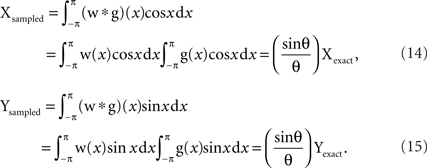Thus VS of sampled spike train is: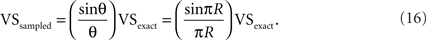Note that VSsampled obtained here does not depend on a specific shape of the spike distribution g(x) whereas the upper and lower bounds discussed in the previous section were obtained only with the von Mises distribution.

We calculated VSsampled for five VSexact values ranging from 0.1 to 0.9 (Figure 3, solid lines). Although VSsampled approaches to 0 when the sampling ratio R = fsignal/fsample increases to 1, it is much more robust to R than the lower bound VSL (Figure 3, dashed lines). Since VSsampled = (sinπRR) VSexact, the “expected error” of VS, defined as eexpected = (VSexact − VSsampled)/VSexact can be calculated as: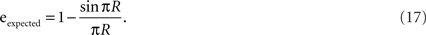Expected errors with several sampling rates are shown in Table 1. Expected error is much smaller than the theoretically calculated maximum error (see also Figure 2), and is less than 2% if the sampling frequency fsample is only 10 times greater than the signal frequency fsignal.

Figure 3 and Table 1 imply that the expected error increases quite slowly with the sampling ratio R for small R values. Using the Taylor expansion sinπR = (πR) − (πR)3/3! + O(R5), the expected error can be calculated as:The approximation eexpected = (πR)2/6 is 99.5% accurate for R < 0.1. This approximation explains the slow increase in the expected error to the sampling ratio.

### Examples from in vivo Recording

In this section, we compare the expected VS errors obtained in the previous subsection with spiking data recorded in vivo. We use data from neurons in the nucleus magnocellularis (NM) and the nucleus laminaris (NL) in the auditory brainstem of owls, chicks, and alligators. These neurons show phase-locked spiking activity and play a key role in sound localization (Carr and Konishi, 1990; Köppl, 1997; Köppl and Carr, 2008; Carr et al., 2009). In our original data set, spike timing was collected with a sampling frequency of 48077 Hz. We downsampled the data with various sampling frequencies and re-calculated VS values (see Materials and Methods). Figure 5 shows the phase-locked activity of eight neurons with best frequencies ranging from 350 to 7000 Hz and with VS ranging from 0.27 to 0.82. In all the neurons shown, VS values decay according to the estimation given as VSsampled = (sinπRR) VSexact (Eq. 16), where the sampling ratio R = fsignal/fsample.

FIGURE 5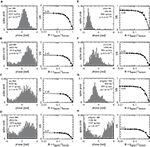Figure 5. Examples from in vivo recording. The left panel in each subfigure is a period histogram showing the spiking probability in each bin. Cell types, stimulus frequency, and the number of spikes recorded are also shown. Note that the number of bins is 50 and therefore the spiking probability in each bin would be 0.02 for non-phase-locked spike trains. The right panel in each subfigure shows the dependence of VS on the sampling ratio R = fsignal/fsample. Open circles indicate vector strengths calculated from the original data sampled at 48077 Hz. Filled circles indicate VS calculated from down-sampled data (see Materials and Methods). Solid lines show VS = (sinπRR) VSexact. (AC) from nucleus magnocellularis (NM) neurons of barn owls, (D) from an NM neuron of a chicken, (E,F) from nucleus laminaris (NL) neurons of chickens (monaural stimulation), (G,H) from NM neurons of alligators.

The above result was entirely consistent with much larger data sets we have tested (Figure 6). Since VSsampled = (sinπRR) VSexact, we can estimate VSexact = (πR/sinπR) VSsampled. We use the data recorded at 48 kHz (original sampling frequency) to obtain the estimate value of VSexact. In Figure 6, we plotted VSsampled from downsampled spike data divided by estimated VSexact. Decay of VSsampled with the sampling ratio R is accurately predicted by the equation VSsampled = (sinπRR) VSexact. When the sampling rate fsample is 20 times as large as the signal frequency fsignal (i.e., R = 0.05), VSsampled can be predicted with a root mean square error of about 1%.

FIGURE 6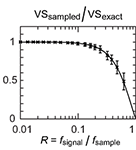Figure 6. Vector strengths calculated from downsampled data (VSsampled) divided by the estimated VSexact calculated from original (non-downsampled) data recorded at 48 kHz. The mean and standard deviation (error bars) of 154 single unit recordings from the auditory brainstem nuclei are shown (68 units in alligators with BFs of 275–1500 Hz and with VS values of 0.20–0.95, 35 units in chicks with BFs of 90–3200 Hz and with VS values of 0.20–0.85, 51 units in owls with BFs of 1400–7000 Hz and with VS values of 0.20–0.77). Four hundred spikes from each unit recording were used to calculate VS values shown. Solid line shows sinπRR with R = fsignal/fsample.

### Sampling Effects on Other Parameters of Circular Distribution

In this section, we examine the sampling effect on several circular statistics other than VS.

#### Mean phase

As we have discussed, the length of the mean vector (=VS) is expected to change as VSsampled = (sinπRR) VSexact by sampling. We did not assume any specific spike detection algorithms in deriving this equation. The direction (phase) of the mean vector, however, strongly depends on the method used in spike discrimination. For example, when peak detection is used to discriminate spikes and detected spike timing tj is assumed to be assigned to the sampling time point nearest to the true peak of the waveform (Figure 1), tj could be before or after the true peak. Assuming that 50% of the spike occurrences are recorded before the true peaks (and, equivalently, the other 50% of the spikes are recorded after the true peaks), the phase of the mean vector is expected to be the same as the true mean.

When threshold detection is used, however, the mean phase could be different from the true direction, because a threshold crossing event is detected only after the waveform crossed the threshold. In this case, mean phase of the recorded spike train is always ahead of the true mean.

Assuming that correct spikes are evenly distributed within the sampling window, the expected shift between the recorded mean phase and the true mean phase can be calculated as: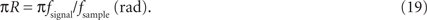From these two different examples, we conclude that the information on the spike discrimination algorithm is necessary to appropriately quantify the sampling effect on the mean phase.

#### Circular standard deviation

Circular standard deviation σ is defined as: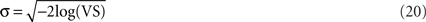(Fisher, 1993). The relationship between the circular standard deviation of the exact distribution and that of the downsampled distribution is calculated as: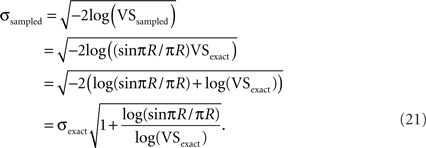Using the Taylor expansions sinπR = (πR) − (πR)3/3! + O(R5), log(1 − x) = −xx2/2 + O(x3), and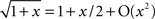, we have: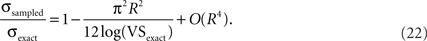This equation indicates that the expected error in circular standard deviation increases sublinearly to the increasing sampling ratio R for small R values (Figure 7A).

FIGURE 7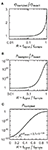Figure 7. Effect of undersampling on the circular standard deviation and significance probability. (A) Increase in circular standard deviation with increasing sampling ratio R (see Eqs. 21 and 22). (B–C) Increase in significance probability with increasing sampling ratio R (see Eqs. 23 and 24). Note the logarithmic scales (abscissa in (B) and ordinates in (B) and (C)). VSexact = 0.5 and N = 1000 are used in this example.

#### Significance probability

Significance probability for VS can be approximated as P = exp(−N(VS)2) with N (>50) being the number of spikes (Fisher, 1993). Defining c = 1 − (sinπRR), the P-values for exact and downsampled data can be related as: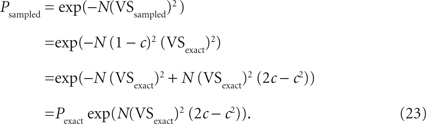Using the Taylor expansions sinπR = (πR) − (πR)3/3! + O(R5), and exp(x) = 1 + x + O(x2), we have: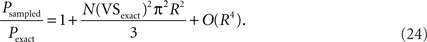Although Eq. 24 indicates that the expected error in the significance probability increases sublinearly to the increasing sampling ratio R for small R values, it is not always practically useful in evaluating P-values for downsampled data. For example, VSexact = 0.5, N = 1000 and R = 0.2 yield Pexact = 2.7 × 10−109 and Psampled = 9.6 × 10−96 (Figure 7B). The significance probability increased more than 1013-fold by downsampling, but Psampled is still far below commonly used significance levels (such as 0.01 or 0.001, see Figure 7C). Thus in examining the significance probability, we suggest using the original equation P = exp(−N(VS)2), instead of Eqs. 23 or 24.

## Discussion

“Any measurement that you make without the knowledge of its uncertainty is completely meaningless” (Lewin, 1999). Although this statement was made originally with physics in mind, it is totally applicable to biological recordings. In this paper we have studied the effect of the length of the sampling window on the measurement of VS, which has been widely used to quantify the degree of phase-locking since it was first introduced to the analysis of neural data 40 years ago (Goldberg and Brown, 1969). We derived theoretical upper and lower bounds for VS with the von Mises distribution (Figures 2, 3 and Table 1). We also calculated the expected errors in VS calculations, assuming random sampling effects but not any specific distribution (Figures 3, 4, and Table 1). The expected error eexpected changes almost linearly to the square of the sampling ratio R (for R < 0.1), indicating that this error does not increase as much as the error in spike timing calculation. Our physiological recordings of auditory brainstem neurons in owls, chicks, and alligators showed that errors in VS can be predicted well by the expected errors we calculated, but not by the theoretical upper and lower bounds of VS, which are several tens to hundred times greater than the expected errors (Figures 4 and 5).

A similar issue was discussed by Bair et al. (1994). They pointed out that the power spectrum of a spike sequence can be corrupted due to the aliasing effect arising from finite sampling intervals. Since VS is the Fourier component of a spike train at the stimulus frequency normalized by the total number of spikes (see, for example, Ashida et al., 2010), VS is nonetheless subject to aliasing, which we refer to as the temporal sampling error. Regarding the Fourier analysis, here we point out the relationship of our results to the Nyquist frequency, which is fsample/2. The Shannon–Nyquist theorem (Shannon, 1949) determines how high a sampling rate is necessary (how many sample points are required) to reconstruct the original analog waveform, assuming that the timing of each sample point is errorless. However, the spike sampling problem, which we have discussed in this paper, corresponds to the question of how high a sampling rate is necessary to accurately calculate a specific Fourier component, assuming that the timing of each sampled spike is subject to measurement error. Therefore, both of these two questions are related to the Fourier analysis, while the latter considers the error in sample timing.

It should be noted that no matter how many spikes are obtained, the temporal sampling error in VS cannot be eliminated. For example, even if spikes in a train are perfectly phase-locked (VSexact = 1), sampling procedure can shift the collected spike timings within the length of the sampling window and therefore calculated vector strength (VSsampled) could be less than 1. Increase in the number of spikes leads to the convergence of VS to the theoretically calculated value of VSsampled but not to VSexact. The way to reduce the temporal sampling error is to increase the sampling rate (or equivalently, to decrease the length of the sampling window). For very precise VS measurement, a sampling rate fsample of 50 times greater than the signal frequency fsignal (i.e., R = 0.02) yields the maximum error of 8% and the expected error of less than 0.1% (Table 1). Practically, however, fsample = 20 × fsignal (i.e., R = 0.05) would suffice because the expected error is still less than 0.5%. When this high sampling frequency is not achievable, fsample = 10 × fsignal (i.e., R = 0.1) might work with an expected error of less than 2%, especially if this amount of error is supposed to be comparable to or less than the errors arising from other sources. If R > 0.1, however, the temporal sampling error will no longer be negligible. In such a case, recorded spike timings need to be corrected to obtain precise VS. Complementary tools for data analysis, such as interpolation (Stoer and Bulirsch, 2002), could improve spike timing measurement and thus reduce the error in VS estimation.

In the preceding analysis and discussion, we implicitly assumed that the frequency and the phase of the reference stimulus can be rigorously determined. Place cells in the rat hippocampus, for example, are known to generate action potentials phase-locked to the internally generated population activity, or the theta oscillation (Harris et al., 2002; Diba and Buzsáki, 2008; Mizuseki et al., 2009). In such cases, frequency and phase of the reference signal need to be calculated from temporally discretized waveforms before phase-locking is quantified. Assuming that conventional Fourier transforms are used to estimate the frequency and the phase, estimation accuracy is governed by the well-known Nyquist–Shannon theory, which requires sampling frequency to be at least twice as high as the signal frequency. Once the reference signal is determined, phase-locking can then be assessed from digitized spike timing data, which is the subject of the present study. Thus in these cases, we still suggest using at least fsample = 10 × fsignal (i.e., R = 0.1), so that the reference signal can be properly estimated and VS can be calculated with an expected error below 2%.

There are multiple sources of variation and errors in VS (Ashida et al., 2010). Some of them are purely biological and the others are more technical. Whereas biological mechanisms of altering VS have been studied intensively (Palmer and Russell, 1986; Weiss and Rose, 1988; Kidd and Weiss, 1990; Rothman et al., 1993; Joris et al., 1994; Joris and Smith, 2008), technical considerations of VS measurement have not yet been fully addressed (e.g., Sullivan and Konishi, 1984; Joris et al., 2006). Although a new metric that can be applied to not only periodic but also aperiodic spiking activity has been proposed recently (Joris et al., 2006), VS is still an intuitive and widely used metric to measure synchrony of periodic spiking activities (Coffey et al., 2006; Köppl and Carr, 2008; Weiss et al., 2009). Therefore systematic investigation on the technical problems of the VS measurement remains practically important.

## Conflict of Interest Statement

The authors declare that the research was conducted in the absence of any commercial or financial relationships that could be construed as a potential conflict of interest.

## Acknowledgments

The authors thank J. L. van Hemmen for his comments on the manuscript. This work was supported by NIH DC00436 to Catherine E. Carr, NIH P30 DC04664 to the University of Maryland Center for the Evolutionary Biology of Hearing.

## References

Ashida, G., Wagner, H., and Carr, C. E. (2010). “Processing of phase-locked spikes and periodic signals,” in Analysis of Parallel Spike Trains, eds S. Grün and S. Rotter (New York: Springer).

Bair, W., Koch, C., Newsome, W., and Britten, K. (1994). Power spectrum analysis of bursting cells in area MT in the behaving monkey. J. Neurosci. 14, 2870–2892.

Carr, C. E., and Friedman, M. A. (1999). Evolution of time coding systems. Neural. Comput. 11, 1–20.

Carr, C. E., and Konishi, M. (1990). A circuit for detection of interaural time differences in the brain stem of the barn owl. J. Neurosci. 10, 3227–3246.

Carr, C. E., and Köppl, C. (2004). Coding interaural time differences at low best frequencies in the barn owl. J. Physiol. (Paris) 98, 99–112.

Carr, C. E., Soares, D., Smolders, J., and Simon, J. Z. (2009). Detection of interaural time differences in the alligator. J. Neurosci. 29, 7978–7982.

Coffey, C. S., Ebert, C. S. Jr, Marshall, A. F., Skaggs, J. D., Falk, S. E., Crocker, W. D., Pearson, J. M., and Fitzpatrick, D. C. (2006). Detection of interaural correlation by neurons in the superior olivary complex, inferior colliculus and auditory cortex of the unanesthetized rabbit. Hear. Res. 221, 1–16.

Diba, K., and Buzsáki, G. (2008). Hippocampal network dynamics constrain the time lag between pyramidal cells across modified environments. J. Neurosci. 28, 13448–13456.

Eggermont, J. J., and Smith, G. M. (1995). Synchrony between single-unit activity and local field potentials in relation to periodicity coding in primary auditory cortex. J. Neurophysiol. 73, 227–245.

Ewert, T. A. S., Vahle-Hinz, C., and Engel, A. K. (2008). High frequency whisker vibration is encoded by phase-locked responses of neurons in the rat’s barrel cortex. J. Neurosci. 28, 5359–5368.

Fisher, N. I. (1993). Statistical Analysis of Circular Data. Cambridge, UK: Cambridge University Press.

Gleich, O., and Narins, P. M. (1988). The phase response of primary auditory afferents in a songbird (Sturnus vulgaris L.). Hear. Res. 32, 81–92.

Goldberg, J. M., and Brown, P. B. (1969). Response of binaural neurons of dog superior olivary complex to dichotic tonal stimuli: some physiological mechanisms of sound localization. J. Neurophysiol. 32, 613–636.

Gray, C., and Singer, W. (1989). Stimulus-specific neuronal oscillations in orientation columns of cat visual cortex. Proc. Natl. Acad. Sci. U.S.A. 86, 1698–1702.

Harris, K. D., Henze, D. A., Hirase, H., Leinekugel, X., Dragoi, G., Czurkó, A., and Buzsáki, G. (2002). Spike train dynamics predicts theta-related phase precision in hippocampal pyramidal cells. Nature 417, 738–741.

Hill, K. G., Stange, G., and Mo, J. (1989). Temporal synchronization in the primary auditory response in the pigeon. Hear. Res. 39, 63–74.

Johnson, D. H. (1980). The relationship between spike rate and synchrony in responses of auditory-nerve fibers to single tones. J. Acoust. Soc. Am. 68, 1115–1122.

Joris, P. X., Carney, L. H., Smith, P. H., and Yin, T. C. T. (1994). Enhancement of neural synchronization in the anteroventral cochlear nucleus. I. Responses to tones at the characteristic frequency. J. Neurophysiol. 71, 1022–1036.

Joris, P. X., Louage, D. H., Cardoen, L., and van der Heijden, M. (2006). Correlation index: a new metric to quantify temporal coding. Hear. Res. 216-217, 19–30.

Joris, P. X., and Smith, P. H. (2008). The volley theory and the spherical cell puzzle. Neuroscience 154, 65–76.

Kawasaki, M., and Guo, Y. X. (1996). Neuronal circuitry for comparison of timing in the electrosensory lateral line lobe of an African wave-type electric fish, Gymnarchus niloticus. J. Neurosci. 16, 380–391.

Kidd, R. C., and Weiss, T. F. (1990). Mechanisms that degrade timing information in the cochlea. Hear. Res. 49, 181–207.

Köppl, C. (1997). Phase locking to high frequencies in the auditory nerve and cochlear nucleus magnocellularis of the barn owl, Tyto alba. J. Neurosci. 17, 3312–3321.

Köppl, C., and Carr, C. E. (2003). Computational diversity in the cochlear nucleus angularis of the barn owl. J. Neurophysiol. 89, 2313–2329.

Köppl, C., and Carr, C. E. (2008). Maps of interaural time difference in the chicken’s brainstem nucleus laminaris. Biol. Cybern. 98, 541–559.

Lewin, W. H. G. (1999). Physics I: classical mechanics. MIT Open Course Ware #8.01. Cambridge, MA: Massachusetts Institute of Technology.

Manley, G. A., Köppl, C., and Yates, G. K. (1997). Activity of primary auditory neurones in the cochlear ganglion of the Emu Dromaius novaehollandiae I: spontaneous discharge, frequency tuning and phase locking. J. Acoust. Soc. Am. 101, 1560–1573.

Mizuseki, K, Sirota, A., Pastalkova, E., and Buzsáki, G. (2009). Theta oscillations provide temporal windows for local circuit computation in the entorhinal-hippocampal loop. Neuron 64, 267–280.

Ogawa, H., Morimoto, K., and Yamashita, Y. (1981). Physiological characteristics of low threshold mechanoreceptor afferent units innervating frog skin. Q. J. Exp. Physiol. 66, 105–116.

Palmer, A. R., and Russell, I. J. (1986). Phase-locking in the cochlear nerve of the guinea-pig and its relation to the receptor potential of inner hair-cells. Hear. Res. 24, 1–15.

Rothman, J. S., Young, E. D., and Manis, P. B. (1993). Convergence of auditory nerve fibers onto bushy cells in the ventral cochlear nucleus: implications of a computational model. J. Neurophysiol. 70, 2562–2583.

Sachs, M. B., and Sinnott, J. M. (1978). Responses to tones of single cells in nucleus magnocellularis and nucleus angularis of the redwing blackbird (Agelaius phoeniceus). J. Comp. Physiol. [A] 126, 347–361.

CrossRef Full Text

Salvi, R. J., Saunders, S. S., Powers, N. L., and Boettcher, F. A. (1992). Discharge patterns of cochlear ganglion neurons in the chicken. J. Comp. Physiol. [A] 170, 227–241.

Sams-Dodd, F., and Capranica, R. R. (1994). Representation of acoustic signals in the eighth nerve of the Tokay gecko: I. Pure tones. Hear. Res. 76, 16–30.

Shannon, C. E. (1949). Communication in the presence of noise. Proc. IRE 37, 10–21.

Smolders, J. W. T., and Klinke, R. (1986). Synchronized responses of primary auditory fiber-populations in Caiman crocodilus (L.) to single tones and clicks. Hear. Res. 24, 89–103.

Stoer, J., and Bulirsch, R. (2002). Introduction to Numerical Analysis, 3rd Edn. New York, NY: Springer.

Stopfer, M., Jayaraman, V., and Laurent G. (2003). Intensity versus identity coding in an olfactory system. Neuron 39, 991–1004.

Sullivan, W. E., and Konishi, M. (1984). Segregation of stimulus phase and intensity coding in the cochlear nucleus of the barn owl. J. Neurosci. 4, 1787–1799.

Weiss, S. A., Preuss, T., and Faber, D. S. (2009). Phase encoding in the Mauthner System: implications in left-right sound source discrimination. J. Neurosci. 29, 3431–3441.

Weiss, T. F., and Rose, C. (1988). Stages of degradation of timing information in the cochlea: a comparison of hair-cell and nerve-fiber responses in the alligator lizard. Hear. Res. 33, 167–174.

Keywords: vector strength, phase-locking, auditory brainstem, sound localization, temporal coding, circular statistics

Citation: Ashida G and Carr CE (2010) Effect of sampling frequency on the measurement of phase-locked action potentials. Front. Neurosci. 4:172. doi: 10.3389/fnins.2010.00172

Received: 27 April 2010; Paper pending published: 23 May 2010;
Accepted: 31 August 2010; Published online: 30 September 2010

Edited by:

Karen A. Moxon, Drexel University, USA

Reviewed by:

Karen A. Moxon, Drexel University, USA
Victor Varga, Institute of Experimental Medicine of the Hungarian Academy of Sciences, Hungary

Copyright: © 2010 Ashida and Carr. This is an open-access article subject to an exclusive license agreement between the authors and the Frontiers Research Foundation, which permits unrestricted use, distribution, and reproduction in any medium, provided the original authors and source are credited.

*Correspondence: Go Ashida, Department of Biology, University of Maryland, College Park, MD 20742-4415, USA. e-mail: ashida@umd.edu